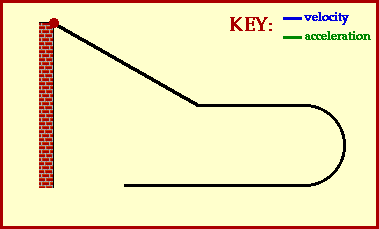## Direction of Acceleration and Velocity

Consider the motion of a Hot Wheels car down an incline, across a level and straight section of track, around a 180-degree curve, and finally along a final straight section of track. Such a motion is depicted in the animation below. The car gains speed while moving down the incline - that is, it accelerates. Along the straight sections of track, the car slows down slightly (due to air resistance forces). Again the car could be described as having an acceleration. Finally, along the 180-degree curve, the car is changing its direction; once more the car is said to have an acceleration due to the change in the direction. Accelerating objects have a changing velocity - either due to a speed change (speeding up or slowing down) or a direction change.This simple animation above depicts some additional information about the car's motion. The velocity and acceleration of the car are depicted by vector arrows. The direction of these arrows are representative of the direction of the velocity and acceleration vectors. Note that the velocity vector is always directed in the same direction which the car is moving. A car moving eastward would be described as having an eastward velocity. And a car moving westward would be described as having a westward velocity.

The direction of the acceleration vector is not so easily determined. As shown in the animation, an eastward heading car can have a westward directed acceleration vector. And a westward heading car can have an eastward directed acceleration vector. So how can the direction of the acceleration vector be determined? A simple rule of thumb for determining the direction of the acceleration is that an object which is slowing down will have an acceleration directed in the direction opposite of its motion. Applying this rule of thumb would lead us to conclude that an eastward heading car can have a westward directed acceleration vector if the car is slowing down.

Be careful when discussing the direction of the acceleration of an object; slow down, apply some thought and use the rule of thumb.

For more information on physical descriptions of motion, visit The Physics Classroom Tutorial. Detailed information is available there on the following topics:

Vectors and Scalars

Speed and Velocity

Acceleration

Describing Motion with Vector Diagrams

Motion Along Inclined Planes